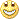[Show all top banners]
 Back to: Kurakani General Discussion Refresh page to view new replieswhere is my mistake ?
 [VIEWED 3655 TIMES] SAVE! for ease of future access.
comingsoonPosted on 02-28-11 12:21 PM     Reply [Subscribe]
Login in to Rate this Post:     0?1) A normal line to the graph of a function f at the point (x,f(x)) is defined to be the line perpendicular to the tangent line at that point . Find the equation of the normal line to the curve  y= ³√x² at the point where x=3
Solution:
the point is (3, 3 2/3).................. [
³√3²=3 2/3]
Now for slope m,
dy/dx=d(x2/3)/dx= 2/3 x-1/3
at poin x=3
2* 3 -1/3/3= 2/(3* 3 1/3) = 2/3 4/3
The line is perpendicular is m=-3 4/3/2
finally the equation of the normal line is
y-32/3/2=
3 4/3/2(x-3)
y=(-3 4/3/2 )x +3 7/2 +3 2/3

Grace_SPosted on 02-28-11 1:32 PM     [Snapshot: 83]     Reply [Subscribe]
Login in to Rate this Post:     0?A simple mathematical error is what I see (happens all the time). See the last step of your calculation. You missed the denominator 2 in the second term.

y-32/3/2=3 4/3/2(x-3)
y=(-3 4/3/2 )x +3 7/2/2 +3 2/3

comingsoonPosted on 02-28-11 1:44 PM     [Snapshot: 117]     Reply [Subscribe]
Login in to Rate this Post:     0?ok thanks actually I have not that error in my paper (typing error)
so that is right equation ?

terobaajePosted on 02-28-11 1:48 PM     [Snapshot: 130]     Reply [Subscribe]
Login in to Rate this Post:     0?math to me is like  "kalo ackcheer bhaisi barabar" lol, wow...das ma das jode bis hunchha timro ra mero oth jde kiss hunchh...thats my math right there lol

Grace_SPosted on 02-28-11 1:56 PM     [Snapshot: 144]     Reply [Subscribe]
Login in to Rate this Post:     0?1) Find the slope of the tangent (lets say m)
2) The slope of the line perpendicular to the tangent would be -1/m
3) Use the slope in the equation: y-y1 = m(x-x1) at the given points.

Good luck!

comingsoonPosted on 02-28-11 2:02 PM     [Snapshot: 163]     Reply [Subscribe]
Login in to Rate this Post:     0?yas , thats i did. But the ans seems like a jokker.comingsoonPosted on 02-28-11 2:08 PM     [Snapshot: 192]     Reply [Subscribe]
Login in to Rate this Post:     0?@Grace_S ,
can you give me rough idea for this question,
when f is defined by f(x)=√x , find a so that f'(a) is three times value of f'(2)
I find f'(2)=1/(2
√2)
so , f'(a)=3/
(2√2)
but I don't know how to find a ? do you have any idea on it?

CountryboyPosted on 03-01-11 8:27 AM     [Snapshot: 499]     Reply [Subscribe]
Login in to Rate this Post:     0?@terobaaje :: that is soo gay daju, made my morning though

Grace_SPosted on 03-02-11 12:35 PM     [Snapshot: 712]     Reply [Subscribe]
Login in to Rate this Post:     0?comingsoon, did you solve for an 'a'? These kinds of problems are like solving mysteries; the more you do, the better is the fun!

ANSPosted on 03-02-11 2:17 PM     [Snapshot: 784]     Reply [Subscribe]
Login in to Rate this Post:     0?Is this correct ??

the point is (3, 3 2/3).................. [³√3²=3 2/3]
Now for slope m,
dy/dx=d(x2/3)/dx= 2/3 x-1/3
at poin x=3
2* 3 -1/3/3= 2*(
3 -1/3* 3 -1) = 2*3 -4/3

The line is perpendicular is m=-2-1*3 4/3

finally the equation of the normal line is
y-32/3=
-2-1*3 4/3(x-3)

y =
-2-1*3 4/3 x + 2-1*32/3 +2-1*3 7/3

OR
y=(-3 4/3/2 )x +
3 2/3 +3 7/3/2